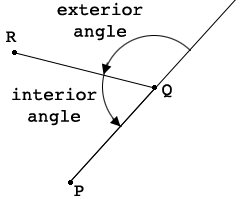SEARCH HOMEMath Central Quandaries & QueriesQuestion from Karan: Prove that a convex polygon cannot have more than three acute internal angles.Hi Karan,

First look at Walter's response to a question about the external angles of a convex polygon where he shows that the sum of the measures of the external angles in $360^{0}.$

In my diagram below $P, Q$ and $R$ are adjacent vertices of a convex polygon where the interior angle is acute.What can you say about the external angle at $Q?$ Suppose there are 4 vertices of the convex polygon where the interior angle is acute.

PennyMath Central is supported by the University of Regina and The Pacific Institute for the Mathematical Sciences.